Mathematical and Physical Journal
for High Schools
Issued by the MATFUND Foundation
 Already signed up? New to KöMaL?

# KöMaL Problems in Physics, May 2020

Show/hide problems of signs:## Problems with sign 'M'

Deadline expired on June 10, 2020.

M. 396. Measure the thickness of a piece of adhesive tape.

(6 pont)

statistics## Problems with sign 'G'

Deadline expired on June 10, 2020.

G. 709. Tarzan targets monkey Maki on a tree. At the moment when the arrow is shot the arrow is aimed at the banana in Maki's hand. At the same moment the frightened monkey drops the banana. What will the arrow hit, if air resistance can be neglected?

(3 pont)

solution (in Hungarian), statistics

G. 710. Anna and Tom are classmates, and they live in a straight street in different houses. Every day they start walking to school at the same time at uniform speeds. Tom lives further from the school, but he is faster, in some time he overtakes Anna. One day Anna would like to meet Tom earlier, so she begins to walk towards Tom. This time they meet five times earlier than they meet usually. By what factor is Tom's speed greater than Anna's speed?

(3 pont)

solution (in Hungarian), statistics

G. 711. A closed underground chamber is connected to the outside world with a chimney. There is water in some parts of the chamber. Determine the pressure at the points $\displaystyle A$, $\displaystyle B$, $\displaystyle C$ and $\displaystyle D$ shown in the figure.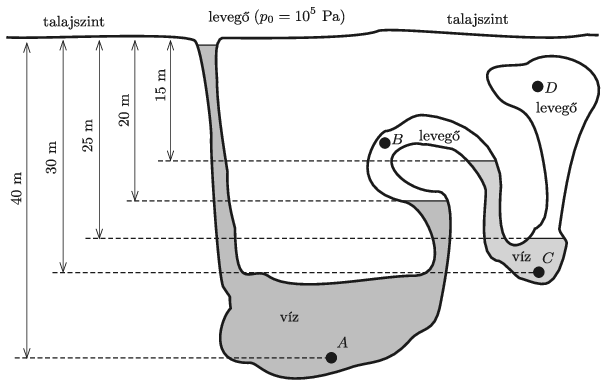(4 pont)

solution (in Hungarian), statistics

G. 712. Statement: At not too small temperature values the molar heat capacity of metals is the same, approximately $\displaystyle 3R=24.9~\rm J/(mol\,K)$, where $\displaystyle R$ is the so called Regnault constant or universal gas constant. Investigate with what percentage accuracy this statement is satisfied for aluminium, gold, silver, copper and iron.

(3 pont)

solution (in Hungarian), statistics## Problems with sign 'P'

Deadline expired on June 10, 2020.

P. 5230. Laminated steel (wrongly called Damascus steel) is made by forging two layers of steel with different carbon content, which like puff pastry, is stretched to double its area and then folded in half. How many times would this process have to be repeated in order to make the thickness of a single layer atomic in size if initially the thickness of the steel was 3 mm?

(3 pont)

solution (in Hungarian), statistics

P. 5231. An apple is held at its stem on three threads of equal length. The threads are alike, they break at the same load. The upper ends of the threads are slowly separated from each other in a horizontal plane so that the angle between any two pairs of threads is the same. The threads are torn when they are (pairwise) just perpendicular to each other. If we were to attach the same apple to two of the same threads and then separate the upper ends of the threads in the same horizontal plane, what angle would the threads make with each other when they tore apart?

(4 pont)

solution (in Hungarian), statistics

P. 5232. The radius of the bottom sphere of a thin-walled, celluloid roly-poly toy is 3 cm. Inside the toy, a 2 cm diameter steel ball was fixed at the bottom. The roly-poly toy is slowly deflected such that the angle between the vertical and its axis of symmetry is $\displaystyle 30^\circ$. What will the angular velocity of the roly-poly toy be at the moment when its axis swings over the vertical position? (Static friction is high enough, the toy does not slip on the ground. Rolling friction and air resistance can be neglected.)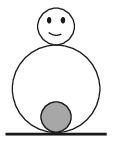(4 pont)

solution (in Hungarian), statistics

P. 5233. A leaf frog wants to jump from the ground onto a leaf, which is horizontally at a distance of $\displaystyle s$ and which is at a height of $\displaystyle h$. In what direction and at what speed does it have to jump in order to do this with the least energy?

(5 pont)

solution (in Hungarian), statistics

P. 5234. A cylindrical container with a vertical axis of symmetry is closed at the top by a piston which is considered to be weightless, as shown in the figure. The container of volume 7 dm$\displaystyle {}^3$ contains no air, only saturated water vapour at a temperature of $\displaystyle T$. How much work is done by pushing down the piston slowly until it reaches the level of the water in the container? During the process, the temperature of the entire system is constant. Calculate and plot the work done as a function of the temperature $\displaystyle T$, where $\displaystyle 100\;{}^\circ\mathrm{C}\le T\le 370\;{}^\circ\mathrm{C}$. (Data on saturated water vapour pressure, density and water density should be taken from tables.)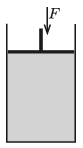(4 pont)

solution (in Hungarian), statistics

P. 5235. A sample of monatomic ideal gas of $\displaystyle n=2$ moles is taken through the process $\displaystyle A\to P\to B$ shown in the figure. The temperature of the gas in the initial state is $\displaystyle T_1=280$ K, and in the final state $\displaystyle T_2=4T_1$. Line segment $\displaystyle AP$ is parallel to axis $\displaystyle V$, the extension of line segment $\displaystyle BC$ passes through the origin, and point $\displaystyle P$ is the midpoint of the line segment $\displaystyle BC$.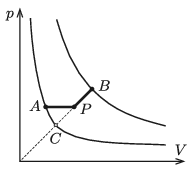$\displaystyle a)$ Determine the temperature of the gas at state $\displaystyle P$.

$\displaystyle b)$ How much heat is absorbed by the gas during process $\displaystyle A\to P\to B$?

(5 pont)

solution (in Hungarian), statistics

P. 5236. A beam of light falls perpendicularly to one side of a glass prism, and it cannot exit at the top part of the other side of the prism, because that part is coated with some reflective material. Below the point of entry the first side of the prism was also coated with a reflective material, so that light cannot escape from the glass in this part either, but is reflected. Finally, the light beam exits the prism on the other side such that its direction of travel is deviated by $\displaystyle 40^\circ$ with respect to the direction of the light beam entering into the prism. What is the angle of the prism $\displaystyle \varphi$ if the refractive index of its material is 1.5?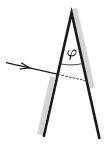(4 pont)

solution (in Hungarian), statistics

P. 5237. A current of 40 mA flows in at point $\displaystyle A$ of the resistor system shown in the figure and flows out at point $\displaystyle B$.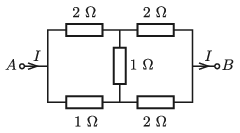$\displaystyle a)$ What is the current through each of the resistors in the system?

$\displaystyle b)$ How much power is dissipated by each resistor?

$\displaystyle c)$ What is the resistance of that single resistor with which we can replace the whole system?

(4 pont)

solution (in Hungarian), statistics

P. 5238. An electric toy car with a mass of $\displaystyle m_0$, and with a load of mass $\displaystyle m$ on it, moves upwards at a constant speed along a slope of elevation angle of $\displaystyle \alpha$. The electric motor, which drives the wheels of radius $\displaystyle r$, can be modelled, when it operates in a steady rate, with a resistor of resistance $\displaystyle R$ which is connected in series with a circuit element, whose voltage $\displaystyle U$ is proportional to the angular velocity of the axle $\displaystyle \omega$ ($\displaystyle U=\gamma \omega$). The current $\displaystyle I$ that flows through it is proportional to the torque $\displaystyle M$ exerted by the axles $\displaystyle (I=M/\gamma)$. The toy car is powered by a battery of e.m.f. $\displaystyle U_0$ and internal resistance of $\displaystyle R_{\mathrm{b}}$.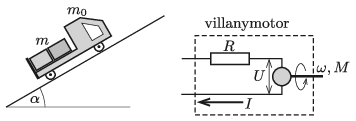Data: $\displaystyle m=300$ g, $\displaystyle \alpha=30^\circ$, $\displaystyle r=2$ cm, $\displaystyle \gamma=1.2$ Vs, $\displaystyle U_0=4{,}5$ V, $\displaystyle R=0{,}8~\Omega$, $\displaystyle R_\text{b}=1.2~\Omega$. (The coefficient of static friction between the wheels and the slope is high enough, so the car does not slip.)

$\displaystyle a)$ At what constant speed does the toy car travel if the mass of the load on it is $\displaystyle m=600$ g?

$\displaystyle b)$ At what load of $\displaystyle m$ will efficiency of the transport of the load be the best? (Efficiency is the ratio of the energy used to lift the load to the energy delivered by the battery.)

(5 pont)

solution (in Hungarian), statistics

P. 5239. At each end of a thin, 21 cm long rigid rod of negligible mass, there is a small point-like body of the same mass. This rod is suspended in the middle by a thin, flexible fibre so that the obtained torsional pendulum has a relatively long period of 600 seconds, which can be measured at small deflections. The pendulum is then hung between two large lead balls, each weighing 600 kg, in the middle. The centres of the lead balls are 70 cm apart. What will the period of the pendulum for small deviations be if the pendulum rod initially lies

$\displaystyle a)$ along the horizontal line segment connecting the centres of the two balls;

$\displaystyle b)$ perpendicularly to the position described in case $\displaystyle a)$?

(6 pont)

solution (in Hungarian), statistics